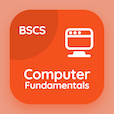Computer Science Online Courses

Digital Logic Design Quizzes

Digital Logic Design Quiz PDF - Complete

# Switching Circuits and Binary Signals Quiz Questions Online p. 49

Learn Switching Circuits and Binary Signals quiz questions and answers, switching circuits and binary signals MCQ with answers PDF 49 to study Digital Logic Design course online. Binary Systems trivia questions, switching circuits and binary signals Multiple Choice Questions (MCQ) for online college degrees. "Switching Circuits and Binary Signals Quiz" PDF Book: d flip flop, binary numbers, counters, lamp handball, switching circuits and binary signals test prep for 2 year computer science degree.

"Voltage operated circuits represent as" MCQ PDF: hexadecimal variables, decimal variables, binary variables, and octal variables to learn online certificate courses. Study binary systems questions and answers to improve problem solving skills for computer and information science.

## Quiz on Switching Circuits & Binary Signals MCQs

MCQ: Voltage operated circuits represent as

Decimal variables
Binary variables
Octal variables

MCQ: Read Only Memory (ROM) simulator is obtained from Random Access Memory (RAM) when it is operated in the

write only mode
write only mode

MCQ: A 12 MHz clock frequency is applied to a cascaded counter containing a modulus-5 counter, a modulus-8 counter, and a modulus-10 counter. The least yield frequency conceivable is?

4input NAND
3input NAND
5input NAND
6input NAND

MCQ: (16)8 in hexadecimal number system is equal to

A
C
E
F

MCQ: A D flip-flop which employs a Positive Going Transition (PGT) clock is in a CLEAR condition. What about the following input behavior that would trigger the state to change?

CLK = NGT, D = 0
CLK = PGT, D = 0
CLOCK NGT, D = 1
CLOCK PGT, D = 1

### More Quizzes from Digital Logic Design Course